# Distance Vector Routing Algorithm | Example

## Routing Algorithms-

• Routing algorithms are meant for determining the routing of packets in a node.
• Routing algorithms are classified as-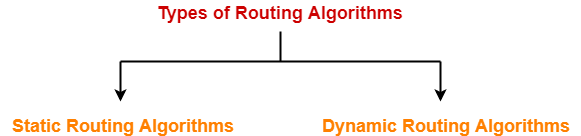1. Static Routing Algorithms
2. Dynamic Routing Algorithms

## Distance Vector Routing Algorithm-

 Distance Vector Routing is a dynamic routing algorithm.

It works in the following steps-

## Step-01:

Each router prepares its routing table. By their local knowledge. each router knows about-

• All the routers present in the network
• Distance to its neighboring routers

## Step-02:

• Each router exchanges its distance vector with its neighboring routers.
• Each router prepares a new routing table using the distance vectors it has obtained from its neighbors.
• This step is repeated for (n-2) times if there are n routers in the network.
• After this, routing tables converge / become stable.

## Distance Vector Routing Example-

Consider-

• There is a network consisting of 4 routers.
• The weights are mentioned on the edges.
• Weights could be distances or costs or delays.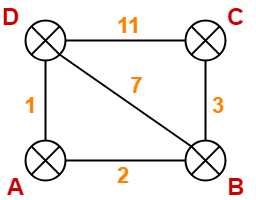## Step-01:

Each router prepares its routing table using its local knowledge.

Routing table prepared by each router is shown below-

### At Router A-

 Destination Distance Next Hop A 0 A B 2 B C ∞ – D 1 D

### At Router B-

 Destination Distance Next Hop A 2 A B 0 B C 3 C D 7 D

### At Router C-

 Destination Distance Next Hop A ∞ – B 3 B C 0 C D 11 D

### At Router D-

 Destination Distance Next Hop A 1 A B 7 B C 11 C D 0 D

## Step-02:

• Each router exchanges its distance vector obtained in Step-01 with its neighbors.
• After exchanging the distance vectors, each router prepares a new routing table.

This is shown below-

### At Router A-

• Router A receives distance vectors from its neighbors B and D.
• Router A prepares a new routing table as-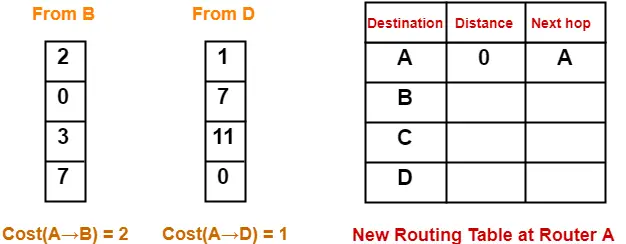• Cost of reaching destination B from router A = min { 2+0 , 1+7 } = 2 via B.
• Cost of reaching destination C from router A = min { 2+3 , 1+11 } = 5 via B.
• Cost of reaching destination D from router A = min { 2+7 , 1+0 } = 1 via D.

### Explanation For Destination B

• Router A can reach the destination router B via its neighbor B or neighbor D.
• It chooses the path which gives the minimum cost.
• Cost of reaching router B from router A via neighbor B = Cost (A→B) + Cost (B→B)= 2 + 0 = 2
• Cost of reaching router B from router A via neighbor D = Cost (A→D) + Cost (D→B) = 1 + 7 = 8
• Since the cost is minimum via neighbor B, so router A chooses the path via B.
• It creates an entry (2, B) for destination B in its new routing table.
• Similarly, we calculate the shortest path distance to each destination router at every router.

Thus, the new routing table at router A is-

 Destination Distance Next Hop A 0 A B 2 B C 5 B D 1 D

### At Router B-

• Router B receives distance vectors from its neighbors A, C and D.
• Router B prepares a new routing table as-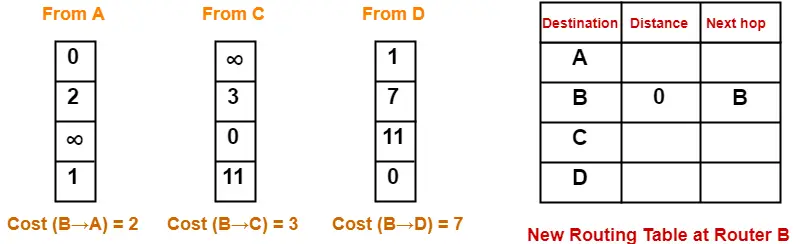• Cost of reaching destination A from router B = min { 2+0 , 3+∞ , 7+1 } = 2 via A.
• Cost of reaching destination C from router B = min { 2+∞ , 3+0 , 7+11 } = 3 via C.
• Cost of reaching destination D from router B = min { 2+1 , 3+11 , 7+0 } = 3 via A.

Thus, the new routing table at router B is-

 Destination Distance Next Hop A 2 A B 0 B C 3 C D 3 A

### At Router C-

• Router C receives distance vectors from its neighbors B and D.
• Router C prepares a new routing table as-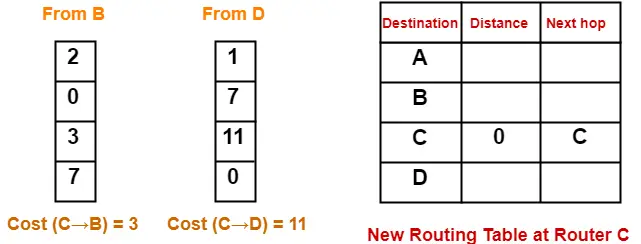• Cost of reaching destination A from router C = min { 3+2 , 11+1 } = 5 via B.
• Cost of reaching destination B from router C = min { 3+0 , 11+7 } = 3 via B.
• Cost of reaching destination D from router C = min { 3+7 , 11+0 } = 10 via B.

Thus, the new routing table at router C is-

 Destination Distance Next Hop A 5 B B 3 B C 0 C D 10 B

### At Router D-

• Router D receives distance vectors from its neighbors A, B and C.
• Router D prepares a new routing table as-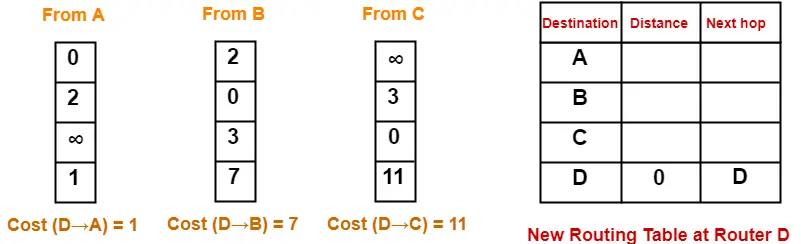• Cost of reaching destination A from router D = min { 1+0 , 7+2 , 11+∞ } = 1 via A.
• Cost of reaching destination B from router D = min { 1+2 , 7+0 , 11+3 } = 3 via A.
• Cost of reaching destination C from router D = min { 1+∞ , 7+3 , 11+0 } = 10 via B.

Thus, the new routing table at router D is-

 Destination Distance Next Hop A 1 A B 3 A C 10 B D 0 D

## Step-03:

• Each router exchanges its distance vector obtained in Step-02 with its neighboring routers.
• After exchanging the distance vectors, each router prepares a new routing table.

This is shown below-

### At Router A-

• Router A receives distance vectors from its neighbors B and D.
• Router A prepares a new routing table as-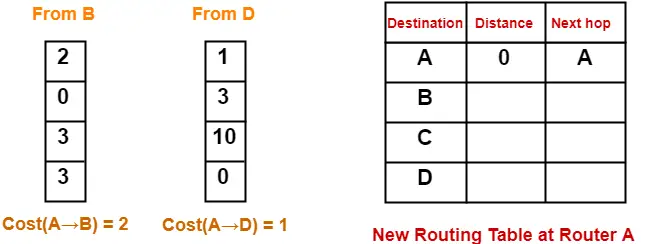• Cost of reaching destination B from router A = min { 2+0 , 1+3 } = 2 via B.
• Cost of reaching destination C from router A = min { 2+3 , 1+10 } = 5 via B.
• Cost of reaching destination D from router A = min { 2+3 , 1+0 } = 1 via D.

Thus, the new routing table at router A is-

 Destination Distance Next Hop A 0 A B 2 B C 5 B D 1 D

### At Router B-

• Router B receives distance vectors from its neighbors A, C and D.
• Router B prepares a new routing table as-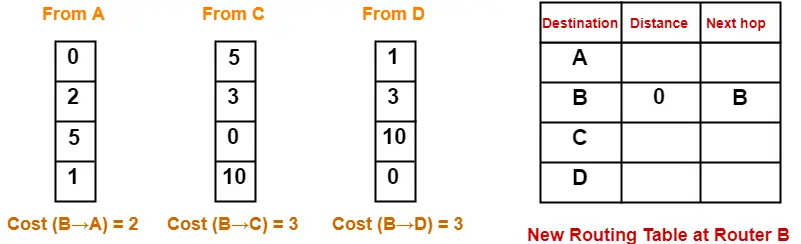• Cost of reaching destination A from router B = min { 2+0 , 3+5 , 3+1 } = 2 via A.
• Cost of reaching destination C from router B = min { 2+5 , 3+0 , 3+10 } = 3 via C.
• Cost of reaching destination D from router B = min { 2+1 , 3+10 , 3+0 } = 3 via A.

Thus, the new routing table at router B is-

 Destination Distance Next Hop A 2 A B 0 B C 3 C D 3 A

### At Router C-

• Router C receives distance vectors from its neighbors B and D.
• Router C prepares a new routing table as-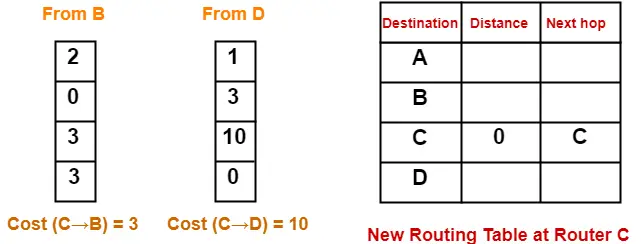• Cost of reaching destination A from router C = min { 3+2 , 10+1 } = 5 via B.
• Cost of reaching destination B from router C = min { 3+0 , 10+3 } = 3 via B.
• Cost of reaching destination D from router C = min { 3+3 , 10+0 } = 6 via B.

Thus, the new routing table at router C is-

 Destination Distance Next Hop A 5 B B 3 B C 0 C D 6 B

### At Router D-

• Router D receives distance vectors from its neighbors A, B and C.
• Router D prepares a new routing table as-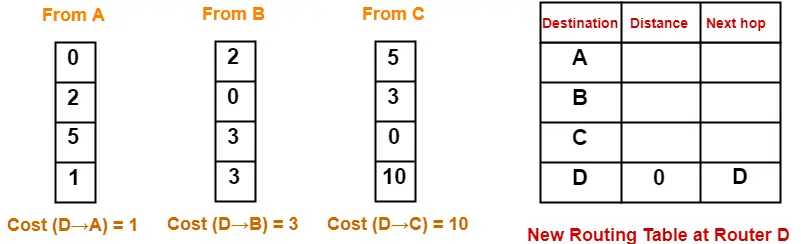• Cost of reaching destination A from router D = min { 1+0 , 3+2 , 10+5 } = 1 via A.
• Cost of reaching destination B from router D = min { 1+2 , 3+0 , 10+3 } = 3 via A.
• Cost of reaching destination C from router D = min { 1+5 , 3+3 , 10+0 } = 6 via A.

Thus, the new routing table at router D is-

 Destination Distance Next Hop A 1 A B 3 A C 6 A D 0 D

These will be the final routing tables at each router.

After routing tables converge (becomes stable),

• Some of the links connecting the routers may never be used.
• In the above example, we can identify the unused links as-

We have-

• The value of next hop in the final routing table of router A suggests that only edges AB and AD are used.
• The value of next hop in the final routing table of router B suggests that only edges BA and BC are used.
• The value of next hop in the final routing table of router C suggests that only edge CB is used.
• The value of next hop in the final routing table of router D suggests that only edge DA is used.

Thus, edges  BD and CD are never used.

## Note-01:

In Distance Vector Routing,

• Only distance vectors are exchanged.
• “Next hop”values are not exchanged.
• This is because it results in exchanging the large amount of data which consumes more bandwidth.

## Note-02:

While preparing a new routing table-

• A router takes into consideration only the distance vectors it has obtained from its neighboring routers.
• It does not take into consideration its old routing table.

## Note-03:

The algorithm is called so because-

• It involves exchanging of distance vectors between the routers.
• Distance vector is nothing but an array of distances.

## Note-04:

• The algorithm keeps on repeating periodically and never stops.
• This is to update the shortest path in case any link goes down or topology changes.

## Note-05:

• Routing tables are prepared total (n-1) times if there are n routers in the given network.
• This is because shortest path between any 2 nodes contains at most n-1 edges if there are n nodes in the graph.

## Note-06:

• Distance Vector Routing suffers from count to infinity problem.
• Distance Vector Routing uses UDP at transport layer.

Get more notes and other study material of Computer Networks.

Watch video lectures by visiting our YouTube channel LearnVidFun.

SummaryArticle Name
Distance Vector Routing Algorithm | Example
Description
Distance Vector Routing Algorithm is a dynamic routing algorithm in computer networks. Distance Vector Routing Algorithm Example. Distance Vector Routing Algorithm is called so because it involves exchanging distance vectors. Each router prepares a routing table and exchange with its neighbors.
Author
Publisher Name
Gate Vidyalay
Publisher Logo